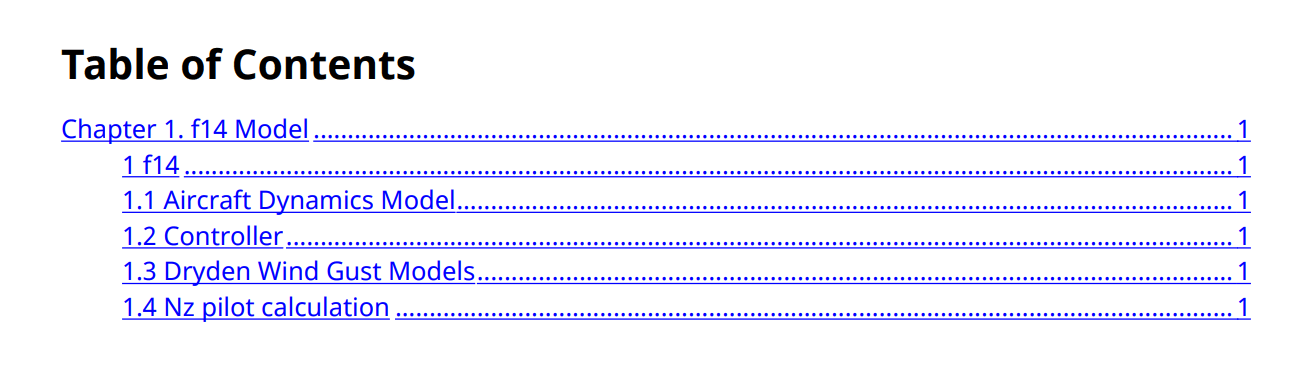slreportgen.utils.HierarchyNumber class

Package: slreportgen.utils

Generate number that represents subsystem position in model hierarchy

Description

Use an object of the slreportgen.utils.HierarchyNumber class to generate numbers that represent the positions of subsystems in a model hierarchy. You can use the numbers to label report elements, such as section titles.

The slreportgen.utils.HierarchyNumber class is a handle class.

Class Attributes

 HandleCompatible true

For information on class attributes, see Class Attributes.

Creation

Description

example

hierarchyNumberObj = slreportgen.utils.HierarchyNumber(source) creates a hierarchy number object for the specified source and sets the Source property to source.

If you specify the source as a Simulink® model, the HierarchyNumber object uses all the uncommented Simulink subsystems and Stateflow® chart diagrams in the model to generate the model hierarchy numbers. Otherwise, the object uses the custom subsystem list that you specify. For example, to generate the hierarchy number of Stateflow chart diagrams in a model, specify a list of the subsystem paths.

Properties

expand all

Model or subsystem, specified as one of these values:

• String scalar or character vector that contains the name of an open or loaded model

• Handle of an open or loaded model

• String array of subsystem paths

• Cell array of subsystem paths or handles

Attributes:

 GetAccess public SetAccess private

expand all

Examples

collapse all

Create an slreportgen.utils.HierarchyNumber object for the slrgex_f14 model and generate the number that represents the position of the Aircraft Dynamics Model subsystem in the model hierarchy.

modelName = "slrgex_f14";
hierNumberObj = slreportgen.utils.HierarchyNumber(modelName);
hierNumber = generateHierarchyNumber(hierNumberObj,"slrgex_f14/Aircraft Dynamics Model")
hierNumber =
"1.1"

This example generates the hierarchy number for each diagram in a model and uses the number in the title of the report section that corresponds to the diagram.

Import the packages so that you do not have to use long, fully-qualified class names.

import mlreportgen.dom.*;
import mlreportgen.report.*;
import slreportgen.finder.*;
import slreportgen.utils.*;

model = "slrgex_f14";

Create a report. Turn off numbering for the report sections.

rpt = Report("output","pdf");
open(rpt);
mlreportgen.report.Section.number(rpt,false);

toc = TableOfContents();
append(rpt,toc);

Create a chapter and turn on chapter numbering.

chapter = Chapter(model + " Model");
chapter.Numbered = true;

Create a diagram finder and a HierarchyNumber object for the model.

finder = DiagramFinder(model);
hierNumberObj = HierarchyNumber(model);

For each found diagram, generate the number that represents the relative position of the diagram in the model hierarchy. Create a section for the diagram and use the generated number in the title. Turn off the default section numbering. Append the section to the chapter.

while hasNext(finder)
result = next(finder);
hierNumber = hierNumberObj.generateHierarchyNumber(result.Path);
title = strcat (hierNumber + " " + result.Name);
section = Section(title);
section.Numbered = false;
append(chapter, section);
end

Append the chapter to the report.

append(rpt,chapter);

Close and view the report.

close(rpt);
rptview(rpt);